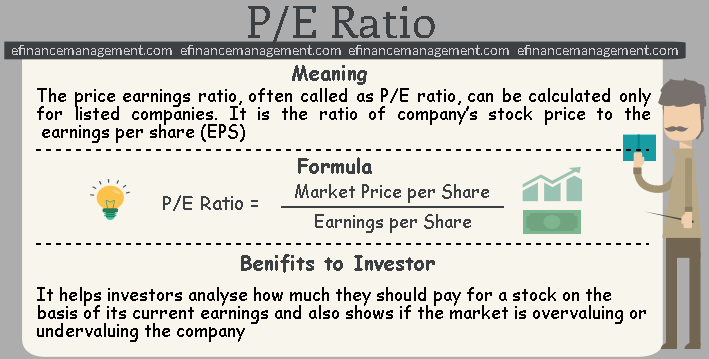# P/E Ratio

## P/E Ratio Meaning

The price-earnings ratio, often called as P/E ratio is the ratio of company’s stock price to the company’s earnings per share. It is a market prospect ratio which is useful in valuing companies. In simple words, P/E ratio is obtained by comparing the market price per share with its relative dollar of earnings per share. The relationship between the two essential parts of this ratio i.e. Market value of the stock and its relative earnings shows what the market is willing to pay for a stock based on its current earnings. Thus, it is also known as the price multiple or the earnings multiple.

## P/E Ratio Valuation

As P/E ratio is the most common measure of how expensive a stock is, it is essential to understand the basis & importance of its valuation.

The two most important components which form the basis of this valuation is:

1. Market value per share and
2. Earnings per share

Along with the above factors, this ratio can also significantly fluctuate depending upon the economic and market conditions.

The following paragraphs will help you understand the importance of such analysis through the P/E ratio formula and calculation.## P/E Ratio Formula

The basic formula to calculate the price-earnings ratio is fairly standard and is as under:

P/E Ratio = Market Price per Share / Earnings per Share
Market Price per Share: Market price per share is the price of each share in the open market or how much it would cost to buy a share of stock.

Earnings per Share (EPS): Earnings per share are total earnings of a company for the year divided by the total number of shares outstanding at the end of the year.

## P/E Ratio Calculation

Let us calculate the price-earnings ratio on the basis of the above formula.

Suppose, the market price per share of QPR Ltd. is Rs.100 and the earnings per share are Rs.25, then the price-earning ratio shall be as follows:

P/E Ratio = Rs.100 (Market Price) / Rs.25 (Earnings)

= 4

This means that the Market price is 4 times the earnings of the company.

## Benefits to Investors

Ratio analysis is very crucial for investment decisions, as it helps the investors to know the real worth of their investment. The P/E ratio is useful in accessing the relative attractiveness of a potential investment. It helps investors analyze how much they should pay for a stock on the basis of its current earnings and also shows if the market is overvaluing or undervaluing the company. It helps in predicting future earnings per share through which the investors evaluate what a stock’s fair market value should be.

## P/E Ratio Analysis

Generally, the pe ratio indicates how many times earnings, the investors are willing to pay for the share. The P/E ratio analysis shows the direct relationship between the market price of the share of a company and its earnings. Hence, if a company’s earnings per share rise; it leads to a rise in its market price, while lower earnings per share indicate a fall in its market price. Thus, these two factors mainly define the real performance and growth of a company.

Similarly, a company with a high pe ratio are often considered to be growth stocks. This indicates higher earnings growth, positive performance in the future and investors are usually willing to pay more for this company’s shares. While on the other hand, a company with a lower pe ratio indicates poor current and future earnings growth, the stock is undervalued, etc. Investing in such company could prove to be a poor investment.

It is important to note that companies with high P/E ratios are more likely to be considered as risky investments than those with lower ones. It is because of the reason that a high P/E ratio signifies high expectations. This ratio is useful only in comparing companies in the same industry. Any such comparisons amongst companies of the different industry would provide an incorrect result and thus, would mislead the investors.

### Example

Let us understand this by an example. Suppose, there are two companies- A Ltd. (belonging to the textile industry) and B Ltd. (belonging to the pharmaceutical industry) with price-earnings ratios 4 and 5 respectively. Also, there is one more company C Ltd. (belonging to the textile industry) with price-earnings ratio 4.5.

Now, while analyzing the price-earnings ratios, one can compare A Ltd. with C Ltd. since they belong to the same industry. As their valuation and growth rates will more or less be alike. One should not or cannot compare either of the two with B Ltd. as it would provide inappropriate results.

Conclusion

The P/E ratio is prominent for the investment valuation indicators. It is because it indicates the expected price of a share based on its earnings. And therefore, the investment community makes the extensive use of this valuation metric.1–3

1.
Investment Valuation Ratios: Price/Earnings Ratio. Investopedia. December 2018. [Source]
2.
What is P/E Ratio? definition and meaning. InvestorWords.com. December 2018. [Source]
3.
Price Earnings P/E Ratio | Analysis | Formula | Example. My Accounting Course. December 2018. [Source]
Last updated on : August 28th, 2019